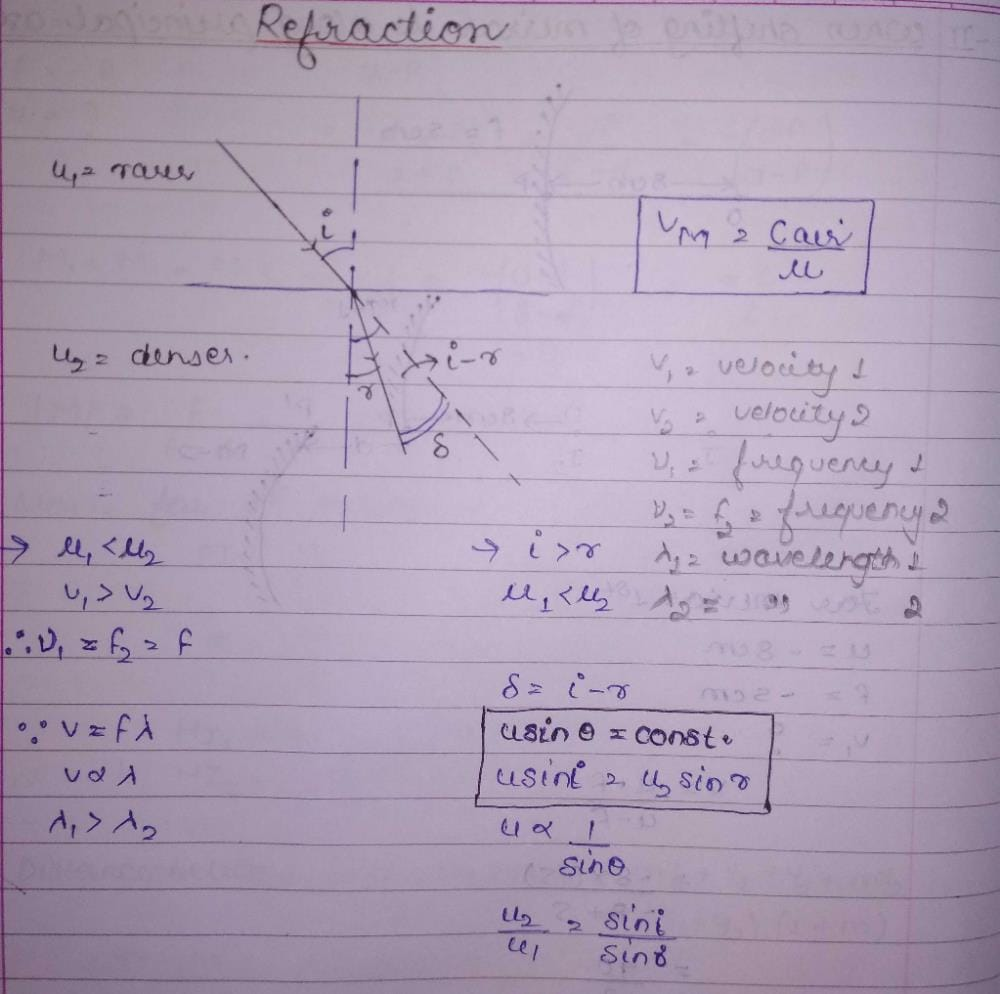When light is refracted into a denser medium,...

### Related TestWhen light is refracted into a denser medium,
• a)
Its wavelength and frequency both increases
• b)
Its wavelength increase but frequency remains unchanged
• c)
Its wavelength decrease but frequency remains unchanged
• d)
Its wavelength and frequency both decreasePioneer AcademyFrequency only depends on the source, i.e. frequency changes only when source changes. So, if light ray travels from one medium to another there will be no change in the frequency because the ray still originates from the same source. For any wave,
Lf = c
where, f = frequency, L = wavelength and c = speed of sound
When light travels from one medium to another there will be a change in its velocity. As a result there will be change in its wavelength since there must be no change in the frequency.

 1 Crore+ students have signed up on EduRev. Have you?Ananya Singh
When light is refracted into a denser medium,a)Its wavelength and frequency both increasesb)Its wavelength increase but frequency remains unchangedc)Its wavelength decrease but frequency remains unchangedd)Its wavelength and frequency both decreaseCorrect answer is option 'C'. Can you explain this answer?View courses related to this question Explore Class 11 courses
 Explore Class 11 coursesView courses related to this questionRam Mohith
Always remember that frequency is only depends on the source, i.e, frequency changes only when source changes. So, if light ray travels from one medium to another there will be no change in the frequency because the ray still originates from the same source. For any wave,
Lf = c
where, f = frequency, L = wavelength and c = speed of sound
When light travels from one medium to another there will be a change in its velocity. As a result there will be change in its wavelength since there must be no change in the frequency.

• ### A bus is moving on a straight road towards north with a uniform speed of 50... more(Scan QR code)